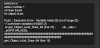# GMS 2.3+another error :/

#### jbug

##### Member
ok so i have another error
i hope someone can explain to me what the error means, i dont quite understand it...
here it is:here is my code for the draw event on oList:
GML:
``````//Draw background
draw_set_color(\$48413b);
draw_roundrect(x, y, x + l_width, y + l_height, 0);
draw_set_color(-1);

//Draw list
var _size = ds_list_size(list);

for (var i = 0; i < _size; i++)
{
//Array data
var _arr = list[| i];
var _name = _arr[PR.NAME];
var _sel = _arr[PR.SELECTED];
var _vals = _arr[PR.VALUES];

//Position to draw
var _x = x + padding;
var _y = y + padding + itemH * i;

//Color
if (i != hoverID) draw_set_color(c_black);

//Draw name
draw_text(_x, _y, _name);

//Draw value
if (_sel > -1)
{
var _val = _vals[_sel];

draw_set_halign(fa_right);
draw_text(x + l_width - padding, _y, _val);
draw_set_halign(fa_left);
}

//Reset color
draw_set_color(c_white);
}``````
also here is the create event where i established some variables:
GML:
``````//Properties
l_width = 400;
l_height = 600;

//GUI
itemH = 48;

//List
list = ds_list_create();

//Variables
hoverID = -1;``````

#### FrostyCat

##### Member
GML:
``var _val = _vals[_sel];``
It says that you are trying to access `_vals` when `_vals` is only of size 2. Arrays are zero-indexed (i.e. the numbering starts from 0), so an array of size 2 only runs 0 through 1. More generally, in anything that's zero-indexed (this includes arrays and lists in GML), something with size N runs 0 through N-1.

#### jbug

##### Member
GML:
``var _val = _vals[_sel];``
It says that you are trying to access `_vals` when `_vals` is only of size 2. Arrays are zero-indexed (i.e. the numbering starts from 0), so an array of size 2 only runs 0 through 1. More generally, in anything that's zero-indexed (this includes arrays and lists in GML), something with size N runs 0 through N-1.
so im a little confused... what did i do wrong?

#### FrostyCat

##### Member
You put incohesive data into `list`, and the error is simply telling you that when the code is unable to use it properly.

Look where those variables are coming from:
GML:
``````   //Array data
var _arr = list[| i];
var _name = _arr[PR.NAME];
var _sel = _arr[PR.SELECTED];
var _vals = _arr[PR.VALUES];``````
If any of the arrays in `list` have a `PR.SELECTED` value of 2 and a `PR.SELECTED` value that is only of size 2, then your error appears. An array of size 2 does NOT have index 2, it only has index 0 and index 1.

Go look at the code responsible for inserting entries into `list` (which is outside of what you've shown on this topic), and make sure that every array it inserts is valid. Don't give it a value in the `PR.SELECTED` position that is equal to or greater than the length of the array in the `PR.VALUES` position.

#### jbug

##### Member
You put incohesive data into `list`, and the error is simply telling you that when the code is unable to use it properly.

Look where those variables are coming from:
GML:
``````   //Array data
var _arr = list[| i];
var _name = _arr[PR.NAME];
var _sel = _arr[PR.SELECTED];
var _vals = _arr[PR.VALUES];``````
If any of the arrays in `list` have a `PR.SELECTED` value of 2 and a `PR.SELECTED` value that is only of size 2, then your error appears. An array of size 2 does NOT have index 2, it only has index 0 and index 1.

Go look at the code responsible for inserting entries into `list` (which is outside of what you've shown on this topic), and make sure that every array it inserts is valid. Don't give it a value in the `PR.SELECTED` position that is equal to or greater than the length of the array in the `PR.VALUES` position.
the is where i used the list variable:
GML:
``````///@arg x
///@arg y
///@arg l_width
///@arg l_height
///@arg LIST_TYPE
function create_list()
{
//Arguments
var _x = argument;
var _y = argument;
var _width = argument;
var _height = argument;
var _type = argument;

//Create list
var _list = instance_create_layer(_x, _y, "Instances", oList);

//Set values
with (_list)
{
l_width = _width;
l_height = _height;
l_type = _type;

//Populate list
switch (_type)
{
case LIST_TYPE.GAME_OPTION:
ds_list_add(list, ["Players", 0, [1, 2, 3, 4]]);
ds_list_add(list, ["Difficulty", 0, ["Easy", "Medium", "Hard", "Insane"]]);
break;
}
}
return _list;
}``````

#### FrostyCat

##### Member
Look in places where the list's arrays are being set away from these default values, especially places where the position `PR.SELECTED` is being set. Walk through code in those places and think how the code can go wrong, e.g. reach 2 or more when there are 2 options.

Once again, in zero-indexing, something of size N runs 0 through N-1.